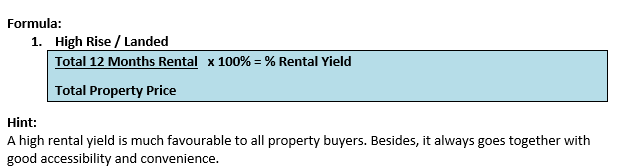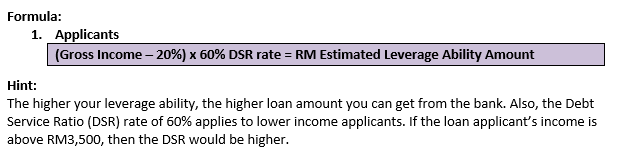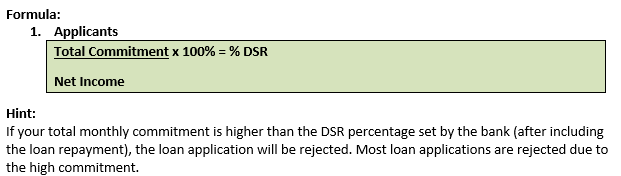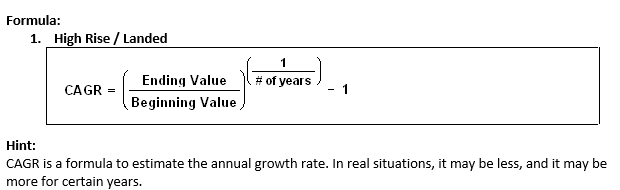8 Property Investment Calculations Every Property Investors Should Learn

# 8 Property Investment Calculations Every Property Investors Should Learn

5955
0
SHAREA successful property investor is not only know how to invest wisely, but good at calculating the numbers. Property investment calculations are another knowledge which every property investors must learn how to calculate the numbers. Here we share you these 8 basic property investment calculations which can aid you in making confident property investments.

### 1. Per Square Foot Price (PSF)

This is the most basic calculation that most people should be aware of! Here’s how you calculate:### 2. Gross Potential Income /  Rental Yield

This is the second most common calculation known by most property buyers. If you’re keen to learn the calculation of a rental yield or how much one can rent it out, here’s how you should answer!### 3. Estimated Leverage Ability

This formula calculates the total amount from your income which can be used for loan purposes.### 4. Debt Service Ratio (DSR)

This formula calculates your current debt. A bank usually determines your loan approval after calculating your DSR, which differs among the banks.### 5. Return On Investment (ROI) / Capital Investment

This refers to the returns of invested capital. It is also what most real estate flipping investors look into since this is their main goal.### 6. Compound Annual Growth Rate (CAGR)

This is the annual growth rate of the investment property purchased at an earlier time.### 7. Return On Equity /  Cash-On-Cash Return (COCR)

This concept is almost the same as the ROI formula, but you can use it to gauge your rental property. The real profit is here for keeper investors.### 8. Gross Rent Multiplier (GRM)

This is one of the tools to decide the rent prices when purchasing a property in a nearby area.Every property investors must learn these calculations and grasp of the details which enables you to make quick, effective decisions.

—WMA Property

SHARE
Previous articleUnderstanding 4 Bumi Attributes in Malaysian Property